# RD Sharma Solutions for Class 11 Chapter 23 - The Straight Lines Exercise 23.18

This exercise deals with equations of lines passing through a given point and making a given angle with a line. Students having any doubts pertaining to the concepts can quickly refer to the RD Sharma Class 11 Maths Solutions, as per their convenience. This is the best resource for any clarifications and helps students prepare confidently for their examinations. The exercise problems are solved by an experienced faculty team having in-depth knowledge about these concepts. In addition, the solutions are prepared, as per the weightage allotted in the board exam. RD Sharma Solutions are available in the pdf format where any student can download easily, from the links provided below.

## Download the pdf of RD Sharma Solutions for Class 11 Maths Exercise 23.18 Chapter 23 – The Straight Lines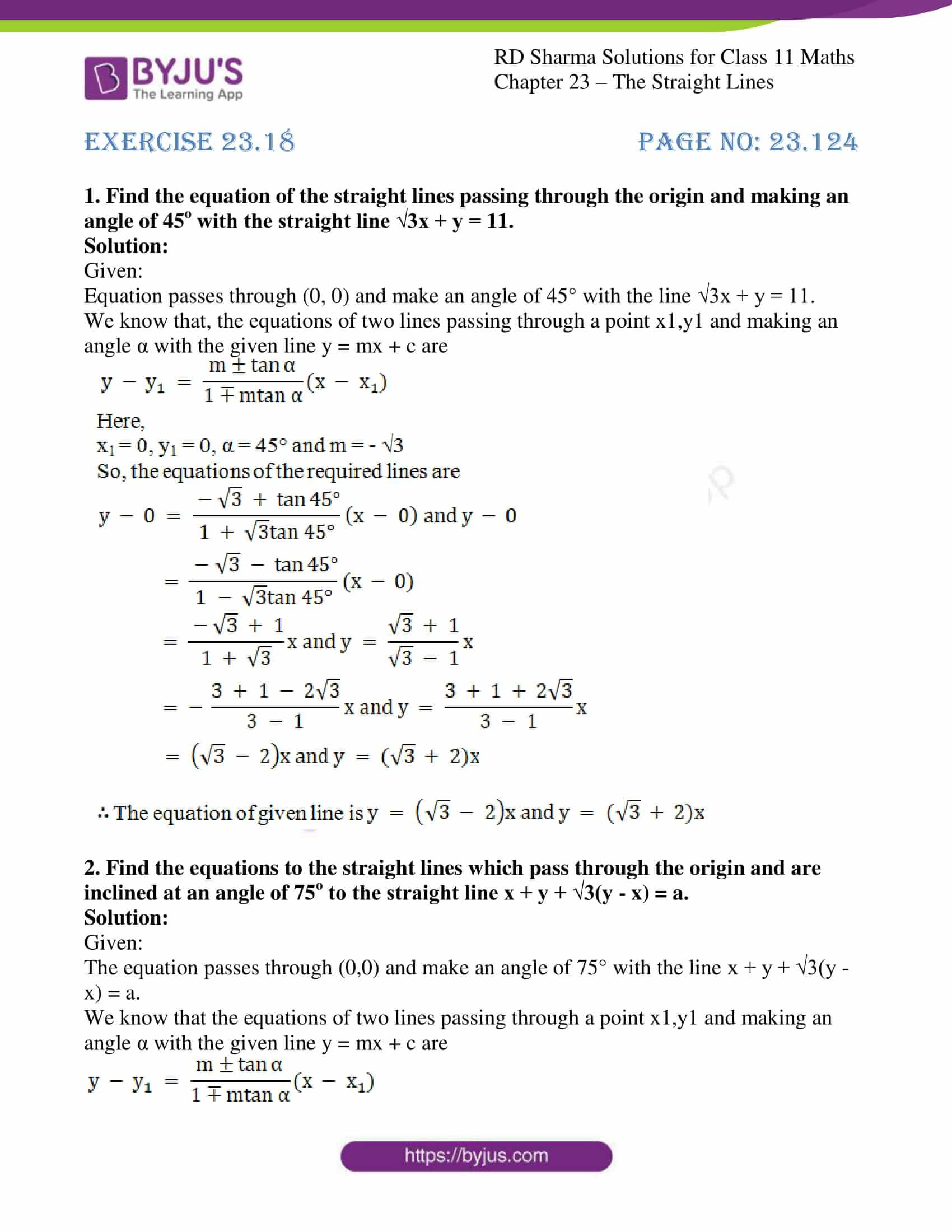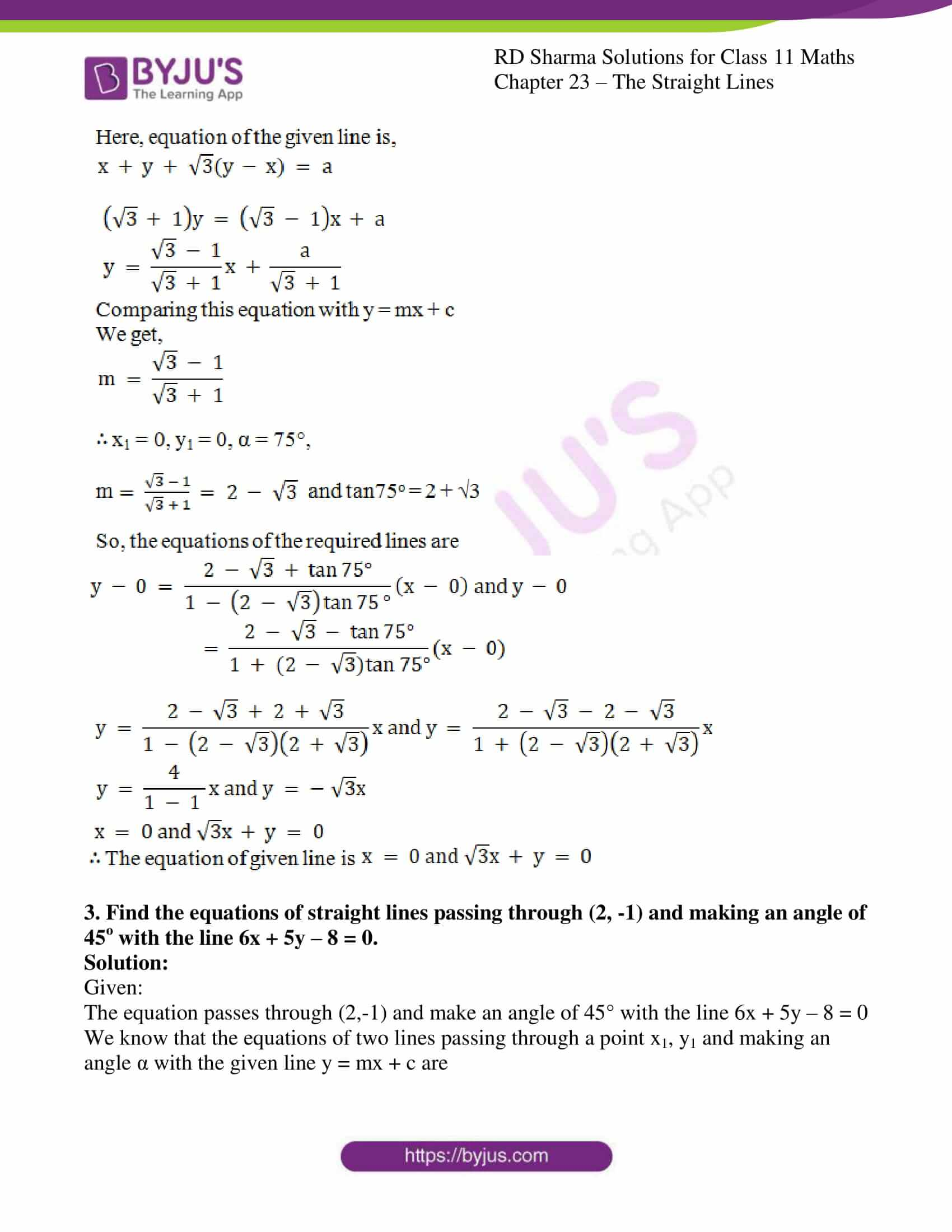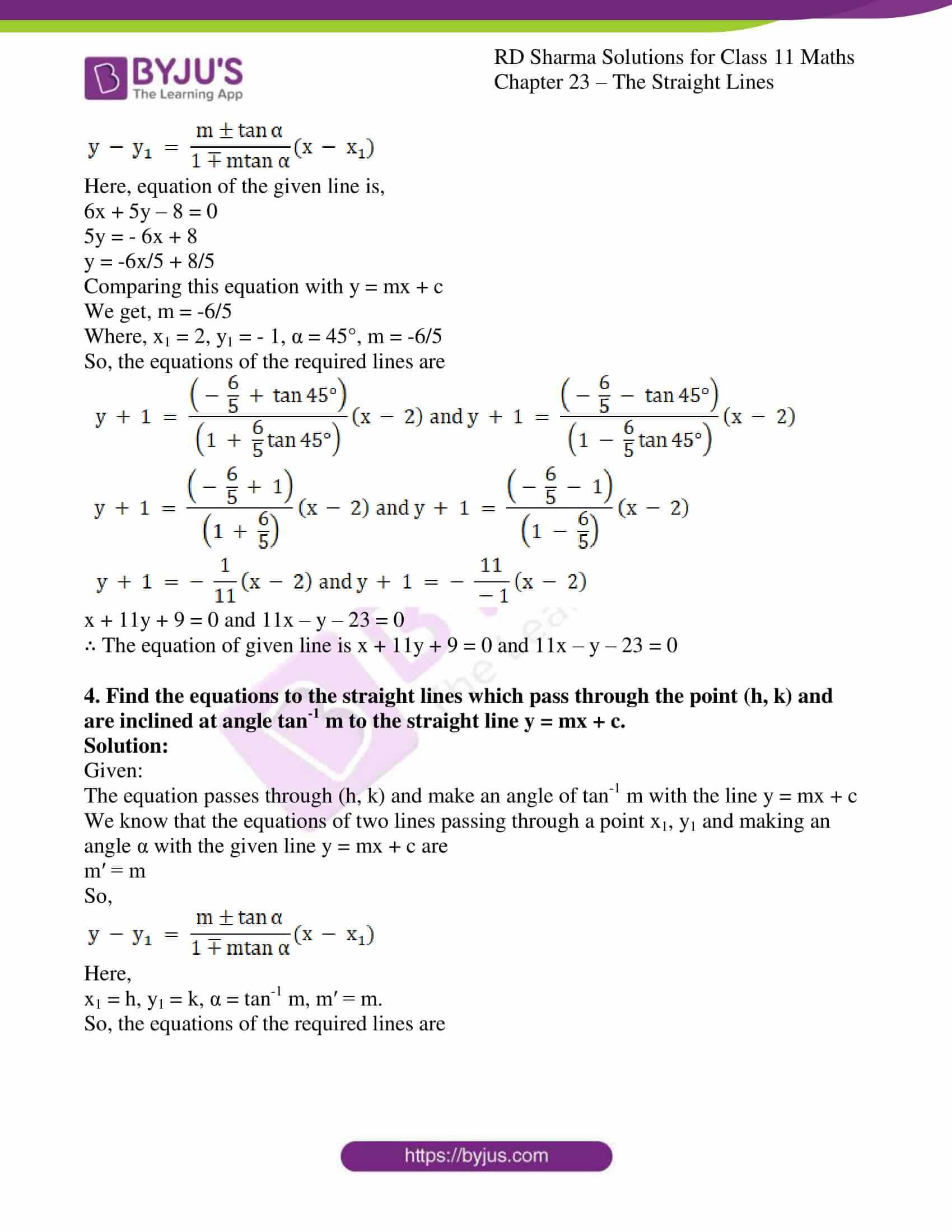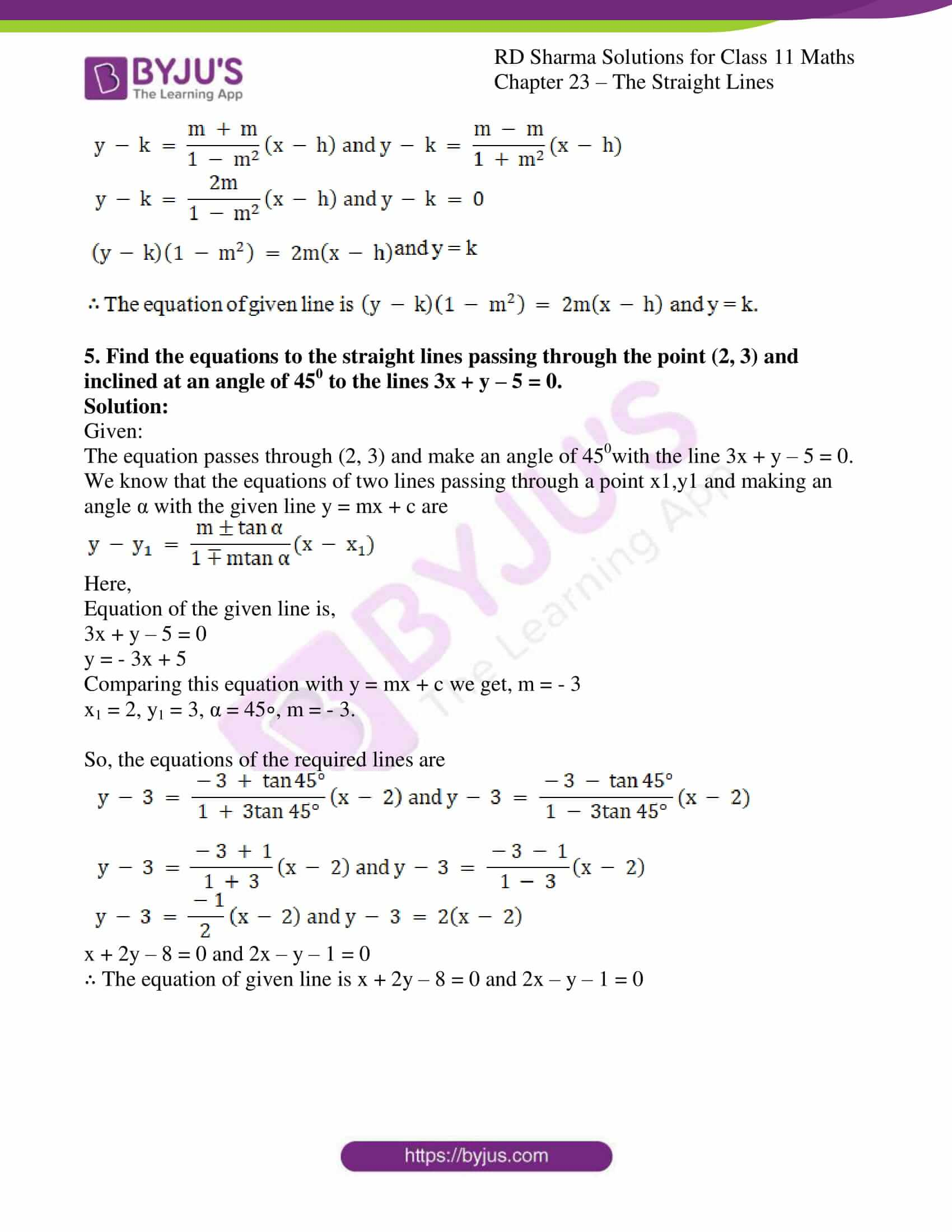### Also, access other exercises of RD Sharma Solutions for Class 11 Maths Chapter 23 – The Straight Lines

Exercise 23.1 Solutions

Exercise 23.2 Solutions

Exercise 23.3 Solutions

Exercise 23.4 Solutions

Exercise 23.5 Solutions

Exercise 23.6 Solutions

Exercise 23.7 Solutions

Exercise 23.8 Solutions

Exercise 23.9 Solutions

Exercise 23.10 Solutions

Exercise 23.11 Solutions

Exercise 23.12 Solutions

Exercise 23.13 Solutions

Exercise 23.14 Solutions

Exercise 23.15 Solutions

Exercise 23.16 Solutions

Exercise 23.17 Solutions

Exercise 23.19 Solutions

### Access answers to RD Sharma Solutions for Class 11 Maths Exercise 23.18 Chapter 23 – The Straight Lines

#### EXERCISE 23.18 PAGE NO: 23.124

1. Find the equation of the straight lines passing through the origin and making an angle of 45o with the straight line √3x + y = 11.

Solution:

Given:

Equation passes through (0, 0) and make an angle of 45° with the line √3x + y = 11.

We know that, the equations of two lines passing through a point x1,y1 and making an angle α with the given line y = mx + c are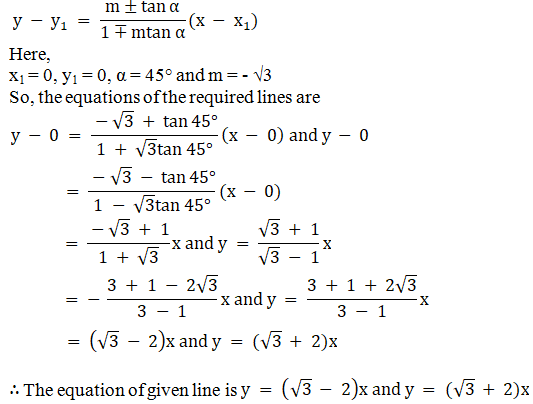2. Find the equations to the straight lines which pass through the origin and are inclined at an angle of 75o to the straight line x + y + √3(y – x) = a.

Solution:

Given:

The equation passes through (0,0) and make an angle of 75° with the line x + y + √3(y – x) = a.

We know that the equations of two lines passing through a point x1,y1 and making an angle α with the given line y = mx + c are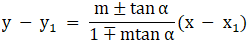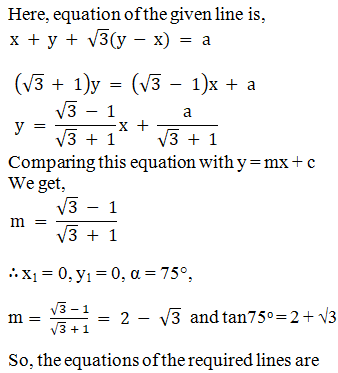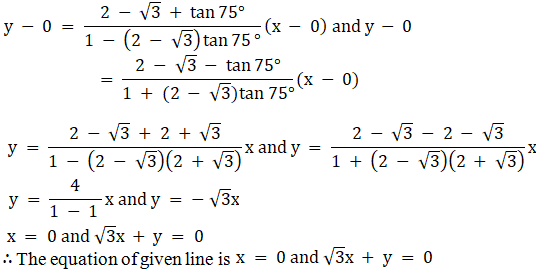3. Find the equations of straight lines passing through (2, -1) and making an angle of 45o with the line 6x + 5y – 8 = 0.

Solution:

Given:

The equation passes through (2,-1) and make an angle of 45° with the line 6x + 5y – 8 = 0

We know that the equations of two lines passing through a point x1, y1 and making an angle α with the given line y = mx + c areHere, equation of the given line is,

6x + 5y – 8 = 0

5y = – 6x + 8

y = -6x/5 + 8/5

Comparing this equation with y = mx + c

We get, m = -6/5

Where, x1 = 2, y1 = – 1, α = 45°, m = -6/5

So, the equations of the required lines are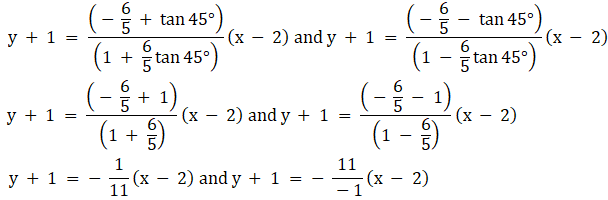x + 11y + 9 = 0 and 11x – y – 23 = 0

∴ The equation of given line is x + 11y + 9 = 0 and 11x – y – 23 = 0

4. Find the equations to the straight lines which pass through the point (h, k) and are inclined at angle tan-1 m to the straight line y = mx + c.

Solution:

Given:

The equation passes through (h, k) and make an angle of tan-1 m with the line y = mx + c

We know that the equations of two lines passing through a point x1, y1 and making an angle α with the given line y = mx + c are

m′ = m

So,Here,

x1 = h, y1 = k, α = tan-1 m, m′ = m.

So, the equations of the required lines are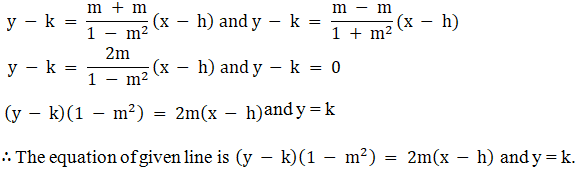5. Find the equations to the straight lines passing through the point (2, 3) and inclined at an angle of 450 to the lines 3x + y – 5 = 0.

Solution:

Given:

The equation passes through (2, 3) and make an angle of 450with the line 3x + y – 5 = 0.

We know that the equations of two lines passing through a point x1,y1 and making an angle α with the given line y = mx + c areHere,

Equation of the given line is,

3x + y – 5 = 0

y = – 3x + 5

Comparing this equation with y = mx + c we get, m = – 3

x1 = 2, y1 = 3, α = 45∘, m = – 3.

So, the equations of the required lines are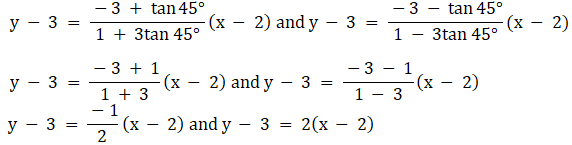x + 2y – 8 = 0 and 2x – y – 1 = 0

∴ The equation of given line is x + 2y – 8 = 0 and 2x – y – 1 = 0